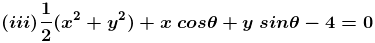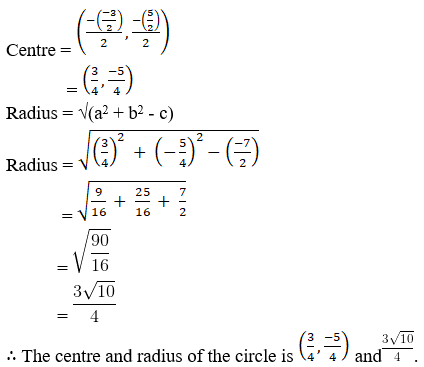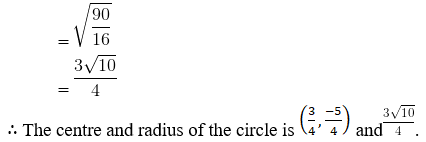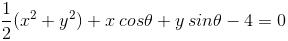• +91 9971497814
• info@interviewmaterial.com

# RD Chapter 24- The Circle Ex-24.2 Interview Questions Answers

### Related Subjects

Question 1 :

Find the coordinates of the centre radius of each of the followingcircle:
(i) x2 + y2 + 6x – 8y – 24 = 0

(ii) 2x2 + 2y2 – 3x + 5y = 7(iv) x2 + y2 – ax – by = 0

(i) x2 + y2 +6x – 8y – 24 = 0

Given:

The equation of thecircle is x2 + y2 + 6x – 8y – 24 = 0 …… (1)

We know that for acircle x2 + y2 + 2ax + 2by + c = 0 …… (2)

Centre = (-a, -b)

So by comparing equation(1) and (2)

Centre = (-6/2,-(-8)/2)

= (-3, 4)

Radius = √(a2 + b2 –c)

= √(32 + 42 –(-24))

= √(9 + 16 + 24)

= √(49)

= 7

The centre ofthe circle is (-3, 4) and the radius is 7.

(ii) 2x2 +2y2 – 3x + 5y = 7

Given:

The equation of thecircle is 2x2 + 2y2 – 3x + 5y = 7 (divide by 2we get)

x2 + y2 –3x/2 + 5y/2 = 7/2

Now, by comparing withthe equation x2 + y2 + 2ax + 2by + c = 0

Centre = (-a, -b)Given:

The equation of thecircle is(Multiply by 2 we get)

x2 + y2 +2x cos θ + 2y sin θ – 8 = 0

By comparing with theequation x2 + y2 + 2ax + 2by + c = 0

Centre = (-a, -b)

= [(-2cos θ)/2 ,(-2sin θ)/2]

= (-cos θ, -sin θ)

Radius = √(a2 + b2 –c)

= √[(-cos θ)2 +(sin θ)2 – (-8)]

= √[cos2 θ+ sin2 θ + 8]

= √[1 + 8]

= √

= 3

The centre andradius of the circle is (-cos θ, -sin θ) and 3.

(iv) x2 + y2 –ax – by = 0

Given:

Equation of the circleis x2 + y2 – ax – by = 0

By comparing with theequation x2 + y2 + 2ax + 2by + c = 0

Centre = (-a, -b)

= (-(-a)/2, -(-b)/2)

= (a/2, b/2)

Radius = √(a2 + b2 –c)

= √[(a/2)2 +(b/2)2]

= √[(a2/4 + b2/4)]

= √[(a2 + b2)/4]

= [√(a2 + b2)]/2

The centre andradius of the circle is (a/2, b/2) and [√(a2 +b2)]/2

Question 2 :
Find the equation of the circle passing through the points :
(i) (5, 7), (8, 1) and (1, 3)
(ii) (1, 2), (3, – 4) and (5, – 6)
(iii) (5, -8), (-2, 9) and (2, 1)
(iv) (0, 0), (-2, 1) and (-3, 2)

(i) (5, 7), (8, 1) and (1, 3)

By using the standardform of the equation of the circle:

x2 + y2 +2ax + 2by + c = 0 ….. (1)

Firstly let us findthe values of a, b and c

Substitute the givenpoint (5, 7) in equation (1), we get

52 + 72 +2a (5) + 2b (7) + c = 0

25 + 49 + 10a + 14b +c = 0

10a + 14b + c + 74 =0….. (2)

By substituting thegiven point (8, 1) in equation (1), we get

82 + 12 +2a (8) + 2b (1) + c = 0

64 + 1 + 16a + 2b + c= 0

16a + 2b + c + 65 =0….. (3)

Substituting the point(1, 3) in equation (1), we get

12 + 32 +2a (1) + 2b (3) + c = 0

1 + 9 + 2a + 6b + c =0

2a + 6b + c + 10 =0….. (4)

Now by simplifying theequations (2), (3), (4) we get the values

a = -29/6, b = -19/6,c = 56/3

Substituting thevalues of a, b, c in equation (1), we get

x2 + y2 +2 (-29/6)x + 2 (-19/6) + 56/3 = 0

x2 + y2 –29x/3 – 19y/3 + 56/3 = 0

3x2 +3y2 – 29x – 19y + 56 = 0

The equation ofthe circle is 3x2 + 3y2 – 29x – 19y + 56 = 0

(ii) (1, 2), (3, – 4) and(5, – 6)

By using the standardform of the equation of the circle:

x2 + y2 +2ax + 2by + c = 0 ….. (1)

Substitute the points(1, 2) in equation (1), we get

12 + 22 +2a (1) + 2b (2) + c = 0

1 + 4 + 2a + 4b + c =0

2a + 4b + c + 5 = 0…..(2)

Substitute the points(3, -4) in equation (1), we get

32 +(- 4)2 + 2a (3) + 2b (- 4) + c = 0

9 + 16 + 6a – 8b + c =0

6a – 8b + c + 25 =0….. (3)

Substitute the points(5, -6) in equation (1), we get

52 +(- 6)2 + 2a (5) + 2b (- 6) + c = 0

25 + 36 + 10a – 12b +c = 0

10a – 12b + c + 61 =0….. (4)

Now by simplifying theequations (2), (3), (4) we get

a = – 11, b = – 2, c =25

Substitute the valuesof a, b and c in equation (1), we get

x2 + y2 +2(- 11)x + 2(- 2) + 25 = 0

x2 + y2 –22x – 4y + 25 = 0

The equation ofthe circle is x2 + y2 – 22x – 4y + 25 = 0

(iii) (5, -8), (-2, 9) and (2, 1)

By using the standardform of the equation of the circle:

x2 + y2 +2ax + 2by + c = 0 ….. (1)

Substitute the point(5, -8) in equation (1), we get

52 +(- 8)2 + 2a(5) + 2b(- 8) + c = 0

25 + 64 + 10a – 16b +c = 0

10a – 16b + c + 89 =0….. (2)

Substitute the points(-2, 9) in equation (1), we get

(- 2)2 +92 + 2a(- 2) + 2b(9) + c = 0

4 + 81 – 4a + 18b + c= 0

-4a + 18b + c + 85 =0….. (3)

Substitute the points(2, 1) in equation (1), we get

22 + 12 +2a(2) + 2b(1) + c = 0

4 + 1 + 4a + 2b + c =0

4a + 2b + c + 5 = 0…..(4)

By simplifyingequations (2), (3), (4) we get

a = 58, b = 24, c = –285.

Now, by substitutingthe values of a, b, c in equation (1), we get

x2 + y2 +2(58)x + 2(24) – 285 = 0

x2 + y2 +116x + 48y – 285 = 0

The equation ofthe circle is x2 + y2 + 116x + 48y – 285 = 0

(iv) (0, 0), (-2, 1) and (-3, 2)

By using the standardform of the equation of the circle:

x2 + y2 +2ax + 2by + c = 0 ….. (1)

Substitute the points(0, 0) in equation (1), we get

02 + 02 +2a(0) + 2b(0) + c = 0

0 + 0 + 0a + 0b + c =0

c = 0….. (2)

Substitute the points(-2, 1) in equation (1), we get

(- 2)2 +12 + 2a(- 2) + 2b(1) + c = 0

4 + 1 – 4a + 2b + c =0

-4a + 2b + c + 5 =0….. (3)

Substitute the points(-3, 2) in equation (1), we get

(- 3)2 +22 + 2a(- 3) + 2b(2) + c = 0

9 + 4 – 6a + 4b + c =0

-6a + 4b + c + 13 =0….. (4)

By simplifying theequations (2), (3), (4) we get

a = -3/2, b = -11/2, c= 0

Now, by substitutingthe values of a, b, c in equation (1), we get

x2 + y2 +2(-3/2)x + 2(-11/2)y + 0 = 0

x2 + y2 –3x – 11y = 0

The equation ofthe circle is x2 + y2 – 3x – 11y = 0

Question 3 : Find the equation of the circle which passes through (3, – 2), (- 2, 0) and has its centre on the line 2x – y = 3.

Given:

The line 2x – y = 3 …(1)

The points (3, -2),(-2, 0)

By using the standardform of the equation of the circle:

x2 + y2 +2ax + 2by + c = 0 ….. (2)

Let us substitute thecentre (-a, -b) in equation (1) we get,

2(- a) – (- b) = 3

-2a + b = 3

2a – b + 3 = 0…… (3)

Now Substitute thegiven points (3, -2) in equation (2), we get

32 +(- 2)2 + 2a(3) + 2b(- 2) + c = 0

9 + 4 + 6a – 4b + c =0

6a – 4b + c + 13 =0….. (4)

Substitute the points(-2, 0) in equation (2), we get

(- 2)2 +02 + 2a(- 2) + 2b(0) + c = 0

4 + 0 – 4a + c = 0

4a – c – 4 = 0….. (5)

By simplifying theequations (3), (4) and (5) we get,

a = 3/2, b = 6, c = 2

Again by substitutingthe values of a, b, c in (2), we get

x2 + y2 +2 (3/2)x + 2 (6)y + 2 = 0

x2 + y2 +3x + 12y + 2 = 0

The equation ofthe circle is x2 + y2 + 3x + 12y + 2 = 0.

Question 4 : Find the equation of the circle which passes through the points (3, 7), (5, 5) and has its centre on line x – 4y = 1.

Given:

The points (3, 7), (5,5)

The line x – 4y = 1….(1)

By using the standardform of the equation of the circle:

x2 + y2 +2ax + 2by + c = 0 ….. (2)

Let us substitute thecentre (-a, -b) in equation (1) we get,

(- a) – 4(- b) = 1

-a + 4b = 1

a – 4b + 1 = 0…… (3)

Substitute the points(3, 7) in equation (2), we get

32 + 72 +2a(3) + 2b(7) + c = 0

9 + 49 + 6a + 14b + c= 0

6a + 14b + c + 58 =0….. (4)

Substitute the points(5, 5) in equation (2), we get

52 + 52 +2a(5) + 2b(5) + c = 0

25 + 25 + 10a + 10b +c = 0

10a + 10b + c + 50 =0….. (5)

By simplifyingequations (3), (4) and (5) we get,

a = 3, b = 1, c = – 90

Now, by substitutingthe values of a, b, c in equation (2), we get

x2 + y2 +2(3)x + 2(1)y – 90 = 0

x2 + y2 +6x + 2y – 90 = 0

The equation ofthe circle is x2 + y2 + 6x + 2y – 90 = 0.

Question 5 : Show that the points (3, -2), (1, 0), (-1, -2) and (1, -4) are con – cyclic.

Given:

The points (3, -2),(1, 0), (-1, -2) and (1, -4)

Let us assume thecircle passes through the points A, B, C.

So by using thestandard form of the equation of the circle:

x2 + y2 +2ax + 2by + c = 0….. (1)

Substitute the pointsA (3, – 2) in equation (1), we get,

32 +(-2)2 + 2a(3) + 2b(-2) + c = 0

9 + 4 + 6a – 4b + c =0

6a – 4b + c + 13 =0….. (2)

Substitute the pointsB (1, 0) in equation (1), we get,

12 + 02 +2a(1) + 2b(0) + c = 0

1 + 2a + c = 0 ……- (3)

Substitute the pointsC (-1, -2) in equation (1), we get,

(- 1)2 +(- 2)2 + 2a(- 1) + 2b(- 2) + c = 0

1 + 4 – 2a – 4b + c =0

5 – 2a – 4b + c = 0

2a + 4b – c – 5 = 0…..(4)

Upon simplifying theequations (2), (3) and (4) we get,

a = – 1, b = 2 and c =1

Substituting thevalues of a, b, c in equation (1), we get

x2 + y2 +2(- 1)x + 2(2)y + 1 = 0

x2 + y2 –2x + 4y + 1 = 0 ….. (5)

Now by substitutingthe point D (1, -4) in equation (5) we get,

12 +(- 4)2 – 2(1) + 4(- 4) + 1

1 + 16 – 2 – 16 + 1

0

The points (3,-2), (1, 0), (-1, -2), (1, -4) are con – cyclic.

Question 6 : Show that the points (5, 5), (6, 4), (- 2, 4) and (7, 1) all lie on a circle, and find its equation, centre, and radius.

Given:

The points (5, 5), (6,4), (- 2, 4) and (7, 1) all lie on a circle.

Let us assume thecircle passes through the points A, B, C.

So by using thestandard form of the equation of the circle:

x2 + y2 +2ax + 2by + c = 0….. (1)

Substituting A (5, 5)in (1), we get,

52 + 52 +2a(5) + 2b(5) + c = 0

25 + 25 + 10a + 10b +c = 0

10a + 10b + c + 50 =0….. (2)

Substitute the pointsB (6, 4) in equation (1), we get,

62 + 42 +2a(6) + 2b(4) + c = 0

36 + 16 + 12a + 8b + c= 0

12a + 8b + c + 52 =0….. (3)

Substitute the point C(-2, 4) in equation (1), we get,

(-2)2 +42 + 2a(-2) + 2b(4) + c = 0

4 + 16 – 4a + 8b + c =0

20 – 4a + 8b + c = 0

4a – 8b – c – 20 =0….. (4)

Upon simplifyingequations (2), (3) and (4) we get,

a = – 2, b = – 1 and c= – 20

Now by substitutingthe values of a, b, c in equation (1), we get

x2 + y2 +2(- 2)x + 2(- 1)y – 20 = 0

x2 + y2 –4x – 2y – 20 = 0 ….. (5)

Substituting D (7, 1)in equation (5) we get,

72 + 12 –4(7) – 2(1) – 20

49 + 1 – 28 – 2 – 20

0

The points (3,-2), (1, 0), (-1, -2), (1, -4) lie on a circle.

Now let us find thecentre and the radius.

We know that for acircle x2 + y2 + 2ax + 2by + c = 0,

Centre = (-a, -b)

Radius = √(a2 + b2 –c)

Comparing equation (5)with equation (1), we get

Centre = [-(-4)/2,-(-2)/2)]

= (2, 1)

Radius = √(22 + 12 –(-20))

= √(25)

= 5

The centre andradius of the circle is (2, 1) and 5.

Question 7 :
Find the equation of the circle which circumscribes the triangle formed by the lines:
(i) x + y + 3 = 0, x – y + 1 = 0 and x = 3
(ii) 2x + y – 3 = 0, x + y – 1 = 0 and 3x + 2y – 5 = 0
(iii) x + y = 2, 3x – 4y = 6 and x – y = 0
(iv) y = x + 2, 3y = 4x and 2y = 3x

(i) x + y + 3 = 0, x – y + 1 = 0 and x = 3

Given:

The lines x + y + 3 =0

x – y + 1 = 0

x = 3

On solving these lineswe get the intersection points A (-2, -1), B (3, 4), C (3, -6)

So by using thestandard form of the equation of the circle:

x2 + y2 +2ax + 2by + c = 0….. (1)

Substitute the points(-2, -1) in equation (1), we get

(- 2)2 +(- 1)2 + 2a(-2) + 2b(-1) + c = 0

4 + 1 – 4a – 2b + c =0

5 – 4a – 2b + c = 0

4a + 2b – c – 5 = 0…..(2)

Substitute the points(3, 4) in equation (1), we get

32 + 42 +2a(3) + 2b(4) + c = 0

9 + 16 + 6a + 8b + c =0

6a + 8b + c + 25 =0….. (3)

Substitute the points(3, -6) in equation (1), we get

32 +(- 6)2 + 2a(3) + 2b(- 6) + c = 0

9 + 36 + 6a – 12b + c= 0

6a – 12b + c + 45 =0….. (4)

Upon simplifyingequations (2), (3), (4) we get

a = – 3, b = 1, c =-15.

Now by substitutingthe values of a, b, c in equation (1), we get

x2 + y2 +2(- 3)x + 2(1)y – 15 = 0

x2 + y2 –6x + 2y – 15 = 0

The equation ofthe circle is x2 + y2 – 6x + 2y – 15 = 0.

(ii) 2x + y – 3 = 0, x + y – 1 = 0 and 3x + 2y – 5 = 0

Given:

The lines 2x + y – 3 =0

x + y – 1 = 0

3x + 2y – 5 = 0

On solving these lineswe get the intersection points A(2, – 1), B(3, – 2), C(1,1)

So by using thestandard form of the equation of the circle:

x2 + y2 +2ax + 2by + c = 0….. (1)

Substitute the points(2, -1) in equation (1), we get

22 +(- 1)2 + 2a(2) + 2b(- 1) + c = 0

4 + 1 + 4a – 2b + c =0

4a – 2b + c + 5 = 0…..(2)

Substitute the points(3, -2) in equation (1), we get

32 +(- 2)2 + 2a(3) + 2b(- 2) + c = 0

9 + 4 + 6a – 4b + c =0

6a – 4b + c + 13 =0….. (3)

Substitute the points(1, 1) in equation (1), we get

12 + 12 +2a(1) + 2b(1) + c = 0

1 + 1 + 2a + 2b + c =0

2a + 2b + c + 2 = 0…..(4)

Upon simplifyingequations (2), (3), (4) we get

a = -13/2, b = -5/2, c= 16

Now by substitutingthe values of a, b, c in equation (1), we get

x2 + y2 +2 (-13/2)x + 2 (-5/2)y + 16 = 0

x2 + y2 –13x – 5y + 16 = 0

The equation ofthe circle is x2 + y2 – 13x – 5y + 16 = 0

(iii) x + y = 2, 3x – 4y = 6 and x – y = 0

Given:

The lines x + y = 2

3x – 4y = 6

x – y = 0

On solving these lineswe get the intersection points A(2,0), B(- 6, – 6), C(1,1)

So by using thestandard form of the equation of the circle:

x2 + y2 +2ax + 2by + c = 0….. (1)

Substitute the points(2, 0) in equation (1), we get

22 + 02 +2a(2) + 2b(0) + c = 0

4 + 4a + c = 0

4a + c + 4 = 0….. (2)

Substitute the point(-6, -6) in equation (1), we get

(- 6)2 +(- 6)2 + 2a(- 6) + 2b(- 6) + c = 0

36 + 36 – 12a – 12b +c = 0

12a + 12b – c – 72 =0….. (3)

Substitute the points(1, 1) in equation (1), we get

12 + 12 +2a(1) + 2b(1) + c = 0

1 + 1 + 2a + 2b + c =0

2a + 2b + c + 2 = 0…..(4)

Upon simplifyingequations (2), (3), (4) we get

a = 2, b = 3, c = –12.

Substituting thevalues of a, b, c in equation (1), we get

x2 + y2 +2(2)x + 2(3)y – 12 = 0

x2 + y2 +4x + 6y – 12 = 0

The equation ofthe circle is x2 + y2 + 4x + 6y – 12 = 0

(iv) y = x + 2, 3y = 4x and 2y = 3x

Given:

The lines y = x + 2

3y = 4x

2y = 3x

On solving these lineswe get the intersection points A(6,8), B(0,0), C(4,6)

So by using thestandard form of the equation of the circle:

x2 + y2 +2ax + 2by + c = 0….. (1)

Substitute the points(6, 8) in equation (1), we get

62 + 82 +2a(6) + 2b(8) + c = 0

36 + 64 + 12a + 16b +c = 0

12a + 16b + c + 100 =0…… (2)

Substitute the points(0, 0) in equation (1), we get

02 + 02 +2a(0) + 2b(0) + c = 0

0 + 0 + 0a + 0b + c =0

c = 0….. (3)

Substitute the points(4, 6) in equation (1), we get

42 + 62 +2a(4) + 2b(6) + c = 0

16 + 36 + 8a + 12b + c= 0

8a + 12b + c + 52 =0….. (4)

Upon simplifyingequations (2), (3), (4) we get

a = – 23, b = 11, c =0

Now by substitutingthe values of a, b, c in equation (1), we get

x2 + y2 +2(- 23)x + 2(11)y + 0 = 0

x2 + y2 –46x + 22y = 0

The equation ofthe circle is x2 + y2 – 46x + 22y = 0

Todays Deals### RD Chapter 24- The Circle Ex-24.2 Contributorskrishan

Name:
Email:

# Latest News# 9000 interview questions in different categories